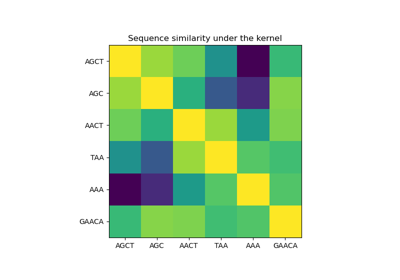# sklearn.gaussian_process.kernels.Hyperparameter¶

class sklearn.gaussian_process.kernels.Hyperparameter[source]

A kernel hyperparameter’s specification in form of a namedtuple.

New in version 0.18.

Attributes
namestr

The name of the hyperparameter. Note that a kernel using a hyperparameter with name “x” must have the attributes self.x and self.x_bounds

value_typestr

The type of the hyperparameter. Currently, only “numeric” hyperparameters are supported.

boundspair of floats >= 0 or “fixed”

The lower and upper bound on the parameter. If n_elements>1, a pair of 1d array with n_elements each may be given alternatively. If the string “fixed” is passed as bounds, the hyperparameter’s value cannot be changed.

n_elementsint, default=1

The number of elements of the hyperparameter value. Defaults to 1, which corresponds to a scalar hyperparameter. n_elements > 1 corresponds to a hyperparameter which is vector-valued, such as, e.g., anisotropic length-scales.

fixedbool, default=None

Whether the value of this hyperparameter is fixed, i.e., cannot be changed during hyperparameter tuning. If None is passed, the “fixed” is derived based on the given bounds.

Methods

 count(self, value, /) Return number of occurrences of value. index(self, value[, start, stop]) Return first index of value.
__init__(self, /, *args, **kwargs)

Initialize self. See help(type(self)) for accurate signature.

__call__(*args, **kwargs)

Call self as a function.

bounds

Alias for field number 2

count(self, value, /)

Return number of occurrences of value.

fixed

Alias for field number 4

index(self, value, start=0, stop=9223372036854775807, /)

Return first index of value.

Raises ValueError if the value is not present.

n_elements

Alias for field number 3

name

Alias for field number 0

value_type

Alias for field number 1

## Examples using sklearn.gaussian_process.kernels.Hyperparameter¶